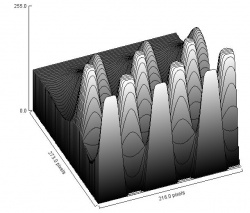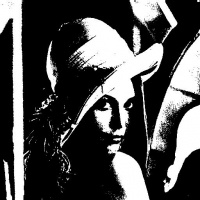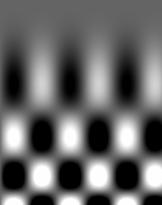##### Tools

This site is devoted to mathematics and its applications. Created and run by Peter Saveliev.

# The topology of a gray scale image

## 1 What we want to get from the image

In binary images objects are either black areas surrounded by white background or white areas surrounded by black background. Similarly, our initial assumption about gray scale images will be that objects are either darker areas surrounded by lighter background or lighter areas surrounded by darker background.

We want to find "objects" in the image. It seems clear that there are 4 light objects on the dark background in the image on the left. Its negative on the right has 4 dark objects on the light background.

One can "binarize" the image but a loss of information is likely:

So, what are those "objects"? The idea comes from considering binary images. Indeed, objects in a binary image are either clusters of adjacent black pixels surrounded by white background, or clusters of adjacent white pixels surrounded by black background, like below.

• Image:
• Its blurry version:

Therefore, objects in a gray scale image should be either connected darker regions surrounded by lighter background, or connected lighter regions surrounded by darker background. On the pixel level this is what we see:

• 4 black objects, one with a hole:
• 3 dark objects, one with a hole:
• Two dark objects each with a hole:

Mathematically, the dark objects are the connected lower level sets and the light objects are the connected supra-level sets of the function that defines the image. The boundaries of the objects are the level curves. Since all of these sets are connected collections of pixels, they will be represented as $0$- and $1$-cycles just as with the binary images.

Note: At this stage the image analysis does not produce unambiguous results because we don't know yet where the boundaries of the objects are.

## 2 Thresholding of gray scale images

The analysis is based on the fact that a gray scale image is nothing but a function of two variables. Higher values mean lighter and lower mean darker:In a binary image $0$ is black and $1$ is white. A gray scale image can represented by an array of numbers between $0$ and $1$. In practice, however, gray scale images are tables of integers. The integers normally run from $0$ (black) to $255$ (white). These numbers together form the gray scale function of the image.

Since we already understand the topology of binary images, our approach will be:

Every image is represented as a combination of binary images.

The idea is illustrated below. Pixels with the gray values below a given threshold will be "black":

Generally, we use thresholding to create binary images from the gray scale image.

Thresholding is the following procedure:

• given a number, called the threshold, $T$ between $0$ and $255$, create a $T$-th image by replacing all the pixels with gray level lower than or equal to $T$ with black ($0$), the rest with white ($1$).

We call these binary images frames

Observe that all thresholds correspond to upper and lower level sets.

Below: the original, and the frames for $T = 50, T = 100, T = 150$.As you raise the threshold, the number of black pixels increases, as in the images above.

This is what happens on the pixel level:

So, the pixel with gray level of $100$ will be white in the first $101$ binary images in the sequence and black in the rest. Now, if we let $T$ run from $0$ to $255$, we end up with a sequence of $256$ binary images.

As an example of topological analysis, let's consider the square image below on the left. A visual inspection of the image reveals that it contains two dark objects represented here as gray scale images of their own:

This information can also be acquired from the analysis of the frames.

The diagram below illustrates the thresholding procedure and creation of the frames. The original gray scale image is at the top. It has 8 levels of gray. Thresholding produces only $4$ (distinct) binary frames because some intermediate levels of gray do not appear in the image. Here $P(i,j)$ stands for the value of the pixel $(i,j)$ in the original, while $B(i,j)$ is the value of that pixel in the binary frame.

Now, the number of objects in each frame is: $1, 2$, and $1$. However, to avoid double-counting, we will only count an object if it does not have an ancestor in the previous frame. Then the total count of objects in the image is: $$1 + 1 + 0 = 2.$$

This is the maximum possible number. One may decide later that one or both of these objects are noise. In the latter case there is only one object – the whole square.

Thresholding creates what we call a filtration of cubical complexes.

## 3 Objects as maxima and minima of the gray scale function

The level of gray of each pixel is given by a number between $0$ and $255$. These numbers together form the gray scale function of the image. An example is on the right.

A grayscale image and its gray scale function:On the pixel level:

The objects in the image correspond to maxima and minima of the gray scale function. This way, as with binary images, they are either connected darker regions surrounded by lighter background, or connected lighter regions surrounded by darker background. (Just like with binary images we have to choose one of these two options. Once again we choose the former.)

Gray scale images are treated as if they have dark objects on light background.

Here, the image has $12$ dark and $8$ light objects. Keep in mind that since the light objects are holes, the ones that touch the border are not counted.

• The gray level function of the original ($1$-dimensional) image:
• The three light objects contained in the image represented as gray scale images of their own:

If the way we capture light objects may be described as “cutting off mountaintops”, the dark objects are captured by "filling in valleys".

## 4 The inclusion tree

Suppose you have a collection of sets that is "nested", i.e., if two sets intersect, one contains the other (cf. Nested Interval Theorem). Then, the collection can be represented by its inclusion tree: an arrow goes from a set to all of its subsets (or vice versa).

A simple example is the collection of all intervals $(-x,x)$. Its inclusion tree has a single branch.

On the opposite end is a collection of disjoint sets...

Now, lower (and upper) level sets of a function are nested, and so are the sets of their connected components. Let's consider some examples.

First, an image – just a blurred black circle:

Second, its level curves, with the lower level sets inside:

Third, they are ordered by inclusion and this fact is reflected in the structure of the tree:

A similar analysis is produced by the negative of this image:

In general, the inclusion trees of an image may be complex:

In a more complex image, not only objects have holes but also there are objects inside the holes. In that case, the inclusion trees for upper and lower level sets, if considered separately, do not contain information about which object has which hole or vice versa. Therefore, in order to capture the topology of the image (with holes), the two trees have to be combined in some way.

That's not hard...

Level sets are the boundaries of the upper and lower level sets. The Jordan Theorem implies that a component of a level set encircles or is encircled by components of other level sets. Then the outer borders of the lower and upper level sets are still ordered and the result is a tree.

An image of a blurred ring, its level curves, and the combined inclusion tree:

This is how the combined inclusion tree might be built from the two inclusion trees:

Exercise: Find the inclusion trees for the images above.

There are drawbacks for this construction:

• The lower level sets are mixed with the upper level sets.
• The gray levels are also mixed.

## 5 A graph representation of the topology of a gray scale image

There is an alternative way to combine the inclusion trees. The upper level tree is turned upside down and attached to the lower level tree – along the aligned layers corresponding to the gray levels:

We will call this the topology graph, just like the one for binary images.

These are the main advantages (as illustrated below):

• The lower and upper inclusion trees remain intact within the graph.
• The graph breaks into layers that coincide with the topology graphs of the frames.

The collection of all possible cycles found in a gray scale image is organized in this graph. Its structure is very similar to that of the topology graph of a binary image: the collections of cycles of the $L$ frames are arranged in $L$ layers within the graph. Each of these cycles encircles a dark component or a light component.

Definition. The nodes of the topology graph of a gray scale image correspond to the cycles (light and dark objects) in the frames of the image and the arrows indicate inclusions as follows:

• there is an arrow from every dark (light) object to a dark (light) component that contains it if they correspond to consecutive gray levels;
• there is an arrow from every dark (light) object to a light (dark) component that contains it if they correspond to the same gray level.

It is important to note here that two identical components may correspond to two different levels of gray and, therefore, may be contained in two consecutive frames. Then, for example, if the gray level of the image is multiplied by $2$, the result is that each layer in the graph is duplicated.

Two examples of images and their topology graphs are given below ($L=3$). The topology graph may be a tree, as in the first image, but generally it isn't, as in the second.

In the first the components are:

• mouth $E$ (black),
• head $B$ (gray),
• eyes $C$ and $D$ (white).

In the second:

• $A$ is the complement of the face and
• $F$ is the complement of the mouth.

## 6 An example of the topology graph

The original gray scale image: 3 dark objects, one with a hole:

The frames:

The cycles in the frames:

The topology graph:

Exercise. Suppose there is an extra black pixel located at (a) left upper, (b) right upper, (c) left lower, (d) right lower corner. Modify the above graph to obtain the topology graph of these images.

## 7 Too many objects?

The nodes of the topology graph represent all "objects and holes" in the image. But, in a sense, there are just too many!

The situation is illustrated below. The first image contains a black circle and the second its blurred version. In either image, there is only a single object.

The level curves of the second image are $255$ concentric circles (rings) with growing - as you move from the center - levels of gray. But it does not contain $256$ objects; it's one dark object!

Even in a simple image as the one below, we have this problem:

There are total of $17$. However not all of them should be counted. In fact, some rectangles are contained in bigger ones.

To have the correct count of objects in a gray scale image, we will follow this uniqueness principle:

If one object contains another, only one of them should be counted.

How can we satisfy it?

The principle is vacuously true for binary images.

For gray scale images, the larger object may be thought of as the background for the smaller one. An extreme case is when the larger object is the whole image. Clearly, it should not be counted.

At this point we would like to say that, with the topology graph, we have captured the topology of the gray scale image. Indeed, the tips of the graph give us all minima and maxima of the gray scale function and, therefore, the "objects" in the image.

But what about the rest of them? We discarded the rest of the nodes following the structure of the graph and nothing else. That's the topological answer. There are infinitely many geometric ways to discard the redundant nodes -- as noise.

Exercise. Draw the topology graph for these images.